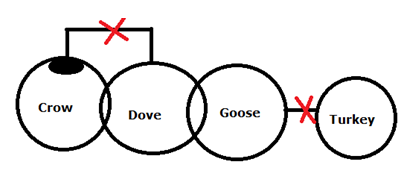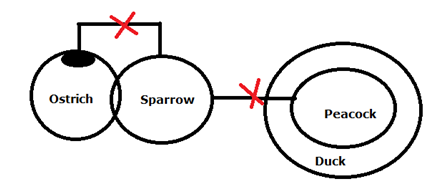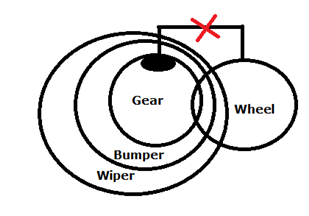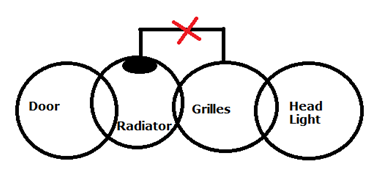# SBI Clerk Prelims Reasoning Ability Questions 2021 (Day-04)

Dear Aspirants, Our IBPS Guide team is providing new series of Reasoning Questions for SBI Clerk Prelims 2021 so the aspirants can practice it on a daily basis. These questions are framed by our skilled experts after understanding your needs thoroughly. Aspirants can practice these new series questions daily to familiarize with the exact exam pattern and make your preparation effective.

Start Quiz

Syllogism

Direction (1-4): Study the following statements and then decide which of the given conclusions logically follows from the given statements disregarding commonly known facts.

a)If Only conclusion I follows.

b)If Only conclusion II follows.

c)If either conclusion I or II follows.

d)If neither conclusion I nor II follows.

e)If both conclusions I and II follow.

1) Statements:

Only a few Crow is Dove

Some Dove is Goose

No Goose is Turkey

Conclusions:

I). All Dove is Turkey

II). Some Crow can be Goose

2) Statements:

Only a few Ostrich is Sparrow

No Sparrow is Peacock

All Peacock is Duck

Conclusions:

I). Some Sparrow being Duck is a possibility

II). Some Ostrich is not Peacock

3) Statements:

Only a few Gears are Wheel

All Gears are Bumper

All Bumpers are Wiper

Conclusions:

I). No Wheel is Bumper

II). All Wipers are Gear

4) Statement

Only a few Radiators are Grilles

Conclusion

I). Some Grilles being Door is a possibility

Misc

5) If in the number 49368512765, the digits at even positions (from the left end) are dropped and the remaining digits are arranged in ascending order from the left end, then which of the following number is second from the right end?

A.1

B.3

C.7

D.8

E.4

Order and ranking

Direction (6-7): Study the following information carefully and answer the below questions.

In a class, there are 50 students get different ranks in the exam and no one is absent for the exam. 14 students are failed in one subject. X ranked 18th from the bottom. Y ranked 17th from the top. Z ranked two ranks above V. V ranked three ranks below X.

6) How many persons are ranked between V and X?

A. One

B. Two

C. Three

D. Four

E. None

7) How many persons are ranked below Z?

A.17

B.18

C.16

D.25

E.30

Inequality

Direction (8-10): In each of the following questions, assuming the given statements to be true, find which of the two conclusions I and II given below them is/are true.

8) Statements:

V ≥ G = H ≤ L > I > P ≥ S

Conclusions:

I). G < L

II). H > P

A. If only conclusion I follows.

B. If only conclusion II follows.

C. If either conclusion I or II follows.

D. If neither conclusion I nor II follows.

E. If both conclusions I and II follow.

9) Statements:

M < K ≤ S = P ≤ T = O > N > R

Conclusions:

I). K < O

II). K = T

A. If only conclusion I follows.

B. If only conclusion II follows.

C. If either conclusion I or II follows.

D. If neither conclusion I nor II follows.

E. If both conclusions I and II follow.

10) Statements:

S ≥ O = V < M ≤ Z < A = X > B

Conclusions:

I). O < Z

II). M > X

A. If only conclusion I follows.

B. If only conclusion II follows.

C. If either conclusion I or II follows.

D. If neither conclusion I nor II follows.

E. If both conclusions I and II follow.

Directions (1-4) :49368512765

438175

134578

Directions (6-7) :

Total-50students

14 students are failed in one subject.

50-14=36 gets ranked.

X ranked 18th from the bottom.

From top X’s rank ->19th rank.

Y ranked 17th from the top.

Z ranked two ranks above V.

V ranked three ranks below X.

V’s rank-22 (from top); Z’s rank-20 (from top)

Directions (8-10) :